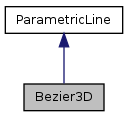QGIS API Documentation  2.6.0-Brighton
Bezier3D Class Reference

Class Bezier3D represents a bezier curve, represented by control points. More...

`#include <Bezier3D.h>`

Inheritance diagram for Bezier3D:[legend]

## Public Member Functions

Bezier3D ()
Default constructor.
Bezier3D (ParametricLine *par, QVector< Point3D * > *controlpoly)
Constructor, par is a pointer to the parent, controlpoly a controlpolygon.
virtual ~Bezier3D ()
Destructor.
virtual void add (ParametricLine *pl)
Do not use this method, since a Bezier curve does not consist of other curves.
virtual void calcFirstDer (float t, Vector3D *v)
Calculates the first derivative and assigns it to v.
virtual void calcSecDer (float t, Vector3D *v)
Calculates the second derivative and assigns it to v.
virtual void calcPoint (float t, Point3D *p)
Calculates the point on the curve and assigns it to p.
virtual void changeDirection ()
changes the order of control points
virtual void remove (int i)
Do not use this method, since a Bezier curve does not consist of other curves.
virtual const Point3DgetControlPoint (int number) const
Returns a control point.
virtual const QVector< Point3D * > * getControlPoly () const
Returns a pointer to the control polygon.
virtual int getDegree () const
Returns the degree of the curve.
virtual ParametricLinegetParent () const
Returns the parent.
virtual void setParent (ParametricLine *par)
Sets the parent.
virtual void setControlPoly (QVector< Point3D * > *cp)
Sets the control polygon.Public Member Functions inherited from ParametricLine
ParametricLine ()
Default constructor.
ParametricLine (ParametricLine *par, QVector< Point3D * > *controlpoly)
Constructor, par is a pointer to the parent object, controlpoly the controlpolygon.
virtual ~ParametricLine ()
Destructor.

## Additional Inherited MembersProtected Attributes inherited from ParametricLine
int mDegree
Degree of the parametric Line.
ParametricLinemParent
Pointer to the parent object.
QVector< Point3D * > * mControlPoly
mControlPoly stores the points of the control polygon

## Detailed Description

Class Bezier3D represents a bezier curve, represented by control points.

Parameter t is running from 0 to 1. The class is capable to calculate the curve point and the first two derivatives belonging to t.

## Constructor & Destructor Documentation

 Bezier3D::Bezier3D ( )
inline

Default constructor.

 Bezier3D::Bezier3D ( ParametricLine * par, QVector< Point3D * > * controlpoly )
inline

Constructor, par is a pointer to the parent, controlpoly a controlpolygon.

Note
not available in python binding
 Bezier3D::~Bezier3D ( )
inlinevirtual

Destructor.

## Member Function Documentation

 void Bezier3D::add ( ParametricLine * pl )
inlinevirtual

Do not use this method, since a Bezier curve does not consist of other curves.

Implements ParametricLine.

 virtual void Bezier3D::calcFirstDer ( float t, Vector3D * v )
virtual

Calculates the first derivative and assigns it to v.

Implements ParametricLine.

 virtual void Bezier3D::calcPoint ( float t, Point3D * p )
virtual

Calculates the point on the curve and assigns it to p.

Implements ParametricLine.

 virtual void Bezier3D::calcSecDer ( float t, Vector3D * v )
virtual

Calculates the second derivative and assigns it to v.

Implements ParametricLine.

 virtual void Bezier3D::changeDirection ( )
virtual

changes the order of control points

Implements ParametricLine.

 const Point3D * Bezier3D::getControlPoint ( int number ) const
inlinevirtual

Returns a control point.

Implements ParametricLine.

 const QVector< Point3D * > * Bezier3D::getControlPoly ( ) const
inlinevirtual

Returns a pointer to the control polygon.

Note
not available in python binding

Implements ParametricLine.

 int Bezier3D::getDegree ( ) const
inlinevirtual

Returns the degree of the curve.

Implements ParametricLine.

 ParametricLine * Bezier3D::getParent ( ) const
inlinevirtual

Returns the parent.

Implements ParametricLine.

 void Bezier3D::remove ( int i )
inlinevirtual

Do not use this method, since a Bezier curve does not consist of other curves.

Implements ParametricLine.

 void Bezier3D::setControlPoly ( QVector< Point3D * > * cp )
inlinevirtual

Sets the control polygon.

Note
not available in python binding

Implements ParametricLine.

 void Bezier3D::setParent ( ParametricLine * par )
inlinevirtual

Sets the parent.

Implements ParametricLine.

The documentation for this class was generated from the following file:
• /tmp/buildd/qgis-2.6.0+wheezy1/src/analysis/interpolation/Bezier3D.h